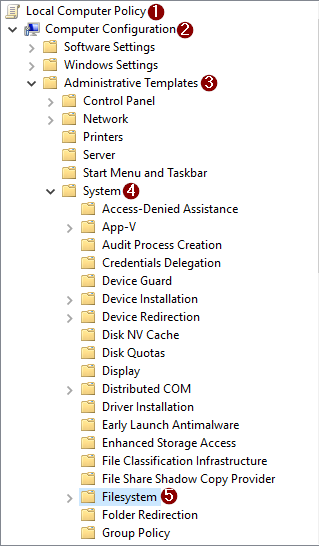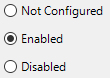Extremely Serious

# Month: August 2018

#### Preface

The following is an example of conditional programming using the key of the map as the condition and the value is the logic to be executed when a particular key was satisfied.

uses java.util.LinkedHashMap

var mainLogicIfRegistry : LinkedHashMap<block(___param : int) : boolean, block()>
= {
\ ___param : int -> 1 == ___param -> \ -> {print("one-0")},
\ ___param : int -> 1 == ___param -> \ -> {print("one-1")},
\ ___param : int -> 2 == ___param -> \ -> {print("two")},
\ ___param : int -> 3 == ___param -> \ -> {print("three")}
}

(1..4).each(\ ___idx ->
mainLogicIfRegistry.Keys.where(\ ___cond -> ___cond(___idx))
.each(\ ___key -> mainLogicIfRegistry.get(___key)())
)

We are using a LinkedHashMap in the example to make the execution based on the order of entries the logic were added in the map. If we are not that concern about the order of logic we can just use a regular map (i.e. HashMap).

#### Inspecting the First Entry in the Map

Let's see how the following first logic was registered in the map.

\ ___param : int -> 1 == ___param -> \ -> {print("one-0")}

The key part of the entry here is the following:

\ ___param : int -> 1 == ___param

The value part of the entry here is the following:

\ -> {print("one-0")}

As we can see both the key and value are lambda expressions and we can repeat the same lambda expression (i.e. but must be different instances) as the key (i.e. as long as you instantiate them separately) since those two are different instances of a block. Knowing this, we can filter the map based on the key (i.e. condition logic) and if it returns true use that key instance to retrieve the value (i.e. logic to execute) from the map.

Note: The lambda expression on the keys must have the same argument signatures. Because the key of the map must be of a consistent type.

Lets interpret the key part, all the lambda expressions of the key requires a parameter (i.e. for consistency of the block instance generated from the lambda expression). So it has one, and is being used to compare to 1. Actually both the first and second entries has the same lambda implementation but with different instances of block. Thus, when we pass in 1, both the first and the second entries will be executed.

Now for the value part, it is just printing the string one-0. To distinguish this from the second entry (i.e. since both the first and second entries has the same lambda expression), the second entry prints the string one-1.

#### Executing the Entries in the Map

Execute a particular entries using the following statement:

mainLogicIfRegistry.Keys.where(\ ___cond -> ___cond(___idx)).each(\ ___key -> mainLogicIfRegistry.get(___key)())

Lets dissect the statement above. We filter the key by executing the logic in the key like the following (i.e. ___idx is a argument of the lambda expression):

mainLogicIfRegistry.Keys.where(\ ___cond -> ___cond(___idx))

Once all the targeted keys were found execute each one like the following:

.each(\ ___key -> mainLogicIfRegistry.get(___key)())

If we execute the code from the preface it will be like this:

one-0
one-1
two
three

As we can see, both the 1 were executed (i.e. one-0 and one-1) followed by the 2 and 3. And since there's no entry for the 4 nothing was executed for it.

For some reason we wanted to abort a current pending merge because maybe of some conflicts. We can use the following command:

git merge --abort

Introduction

A tool for managing parallel Versions of multiple Software Development Kits on a windows based system.

Installation via Short Script

Invoke-WebRequest -Uri 'https://raw.githubusercontent.com/flofreud/posh-gvm/master/GetPoshGvm.ps1' | iex

Usage

Displaying the tool capability using the following:

gvm [help]

Useful Commands

 Command Description list Lists the available, installed and default versions of the candidate. install Downloads and installs a particular candidate version (i.e. will default to latest if version is not specified) use Uses a particular candidate version that is not the default. default Sets a default version to use of a particular candidate. current Displays the current active candidates version. uninstall Uninstalls a particular candidate version.
1. Hit the windows key.
2. Type gpedit.msc (i.e. Microsoft Common Console Document) and Run as administrator.
3. Navigate to Local Computer Policy > Computer Configuration > Administrative Templates > System > Filesystem.4. On the right pane double click Enable Win32 long paths.
5. Select the Enabled radio button.6. Click the OK button.